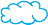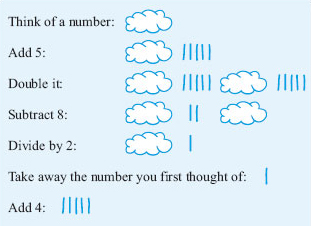Starting with maths: Patterns and formulas

This free course is available to start right now. Review the full course description and key learning outcomes and create an account and enrol if you want a free statement of participation.

Free course

# 5 Your own formulas

## 5.1 Number tricks

In the last section, you worked out some formulas that could be used in a spreadsheet. Section 5 gives you some more practice in deriving formulas both by looking at some number tricks and rearranging some existing formulas.

### Activity 13: Think of a number

Try this number puzzle. (If you use your calculator, remember to press the equals sign after each instruction.)

‘Think of a number. Add 5. Double it. Subtract 8. Divide by 2. Take away the number you first thought of. Add 4.’

Now if 1 represents the letter ‘A’; 2 represents the letter ‘B’; 3 represents the letter ‘C’; and so on, work out the letter represented by your answer and write down the name of an animal beginning with this letter.

#### Discussion

If the number 3 were chosen initially, the instructions work out as follows.

 Add 5: 3 + 5 = 8 Double it: 8 × 2 = 16 Subtract 8: 16 – 8 = 8 Divide by 2: 8 ÷ 2 = 4 Take away number: 4 − 3 = 1 Add 4: 1 + 4 = 5

You will find that you always get 5, whatever number you start with. This gives the letter E. Did you choose an elephant?

To see how this trick works, read through the instructions below. Because we do not know what number you have thought of, we have replaced the number by a thought bubble like this:Figure 15

This shows that the numerical answer is always five – it does not depend on which number was chosen first of all. So the letter of the alphabet chosen is E. There are not many animals with names beginning with E and most people do think of an elephant first, but you might be unlucky if someone chooses an emu or an eel!

Rather than using a cloud to represent the number and explaining the trick pictorially, you could write down expressions for the answer at each stage and use either a word (for example, number) or a letter (for example, N) to represent the number. The trick can then be written as follows.

Instruction Expression
Think of a number n
Add 5 n + 5
Double it n + 5 + n + 5 = 2 × n + 10
Subtract 8 2 × n + 10 – 8 = 2 × n + 2
Divide by 2 (2 × n ÷ 2) + (2 ÷ 2) = n + 1
Take away the number you first thought of n + 1 − n = 1
Add 4 1 + 4 = 5

### Activity 15: Another number trick

Try the following trick several times. Think of a number between 1 and 10. This will work with numbers greater than 10, but the restriction is to keep the arithmetic manageable. Multiply by 4. Add 6. Divide by 2. Subtract 3. Divide by 2, and your answer is?

Write down the number you first thought of and your answer. What do you notice? Can you explain why this happens, either by using a diagram or by writing down the expressions for the answer at each stage?

#### Discussion

You should find that this time the answer is always the number you chose at the start. The expressions for the answer at each stage are shown below.

Instruction Expression
Think of a number between 1 and 10 n
Multiply by 4 4 × n
Add 6 4 × n + 6
Divide by 2 (4 × n ÷ 2) + (6 ÷ 2) = 2 × n + 3
Subtract 3 2 × n + 3 – 3 = 2 × n
Divide by 2 2 × n ÷ 2 = n

Try making up your own number tricks. What makes a good trick?

Y162_2

### Take your learning further

Making the decision to study can be a big step, which is why you'll want a trusted University. The Open University has 50 years’ experience delivering flexible learning and 170,000 students are studying with us right now. Take a look at all Open University courses.

If you are new to university level study, find out more about the types of qualifications we offer, including our entry level Access courses and Certificates.

Not ready for University study then browse over 900 free courses on OpenLearn and sign up to our newsletter to hear about new free courses as they are released.

Every year, thousands of students decide to study with The Open University. With over 120 qualifications, we’ve got the right course for you.

Request an Open University prospectus